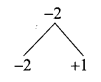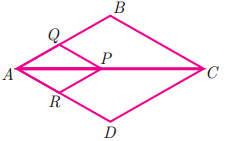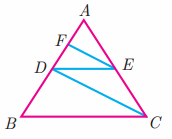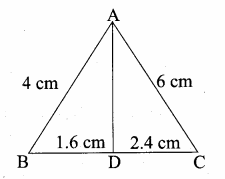## Ex 4.2 | Aster Classes

Tamilnadu, Samacheer, Kalvi, 10th, sslc, Maths, Solutions, Chapter 4, Algebra, Ex 4.2,

Question 1.
In ∆ABC, D and E are points on the sides AB and AC respectively such that DE || BC
(i) If ADDB = 34 and AC = 15 cm find AE.
(ii) If AD = 8x – 7 , DB = 5x – 3 , AE = 4x – 3 and EC = 3x – 1, find the value of x.
Solution:
(i) Let AE be x
∴ EC = 15 – x
In ∆ABC we have DE || BC
By Basic proportionality theorem, we have

34 = x15−x
4x = 3 (15 – x)
4x = 45 – 3x
7x = 45 ⇒ x = 457 = 6.43
The value of x = 6.43

(ii) Given AD = 8x – 7; BD = 5x – 3; AE = 4x – 3; EC = 3x – 1
In ∆ABC we have DE || BC
By Basic proportionality theorem
8x−75x−3 = 4x−33x−1

(8x – 7) (3x – 1) = (4x – 3) (5x – 3)
24x2 – 8x – 21x + 7 = 20x2 – 12x – 15x + 9
24x2 – 20x2 – 29x + 27x + 7 – 9 = 0
4x2 – 2x – 2 = 0
2x2 – x – 1 = 0 (Divided by 2)2x2 – 2x + x – 1 = 0
2x(x -1) + 1 (x – 1) = 0
(x – 1) (2x + 1) = 0
x – 1 = 0 or 2x + 1 = 0
x = 1 or 2x = -1 ⇒ x = – 12 (Negative value will be omitted)
The value of x = 1

Question 2.
ABCD is a trapezium in which AB || DC and P,Q are points on AD and BC respectively, such that PQ || DC if PD = 18 cm, BQ
Solution:
Join AC intersecting PQ at S.
Let AP be x
∴ AD = x + 18
In the ∆ABC, QS || AB
By basic proportionality theorem.

ASSC = BQQC
ASSC = 3515 ………(1)
In the ∆ACD; PS || DC
By basic proportionality theorem.
ASSC = APPD
ASSC = x18 ………..(2)
From (1) and (2) we get
3515 = x18
15x = 35 × 18 ⇒ x = 35×1815 = 42
= 42 + 18 = 60
The value of AD = 60 cm

Question 3.
In ∆ABC, D and E are points on the sides AB and AC respectively. For each of the following cases show that DE || BC.
(i) AB = 12 cm, AD = 8 cm, AE = 12 cm and AC = 18 cm.
(ii) AB = 5.6 cm, AD = 1.4 cm, AC = 7.2 cm and AE = 1.8 cm.
Solution:
(i) Here AB = 12 cm; BD =12 – 8 = 4 cm; AE =12 cm; EC = 18 – 12 = 6 cm

∴ ADDB = 84 = 2
AEEC = 126 = 2
By converse of basic proportionality theorem DE || BC

(ii) Here AB = 5.6 cm; AD = 1.4 cm;
= 5.6 – 1.4 = 4.2

AC = 7.2 cm; AE = 1.8 cm
EC = AC – AE
= 7.2 – 1.8
EC = 5.4 cm
AEEC = 1.85.4 = 13
By converse of basic proportionality theorem DE || BC

Question 4.
In fig. if PQ || BC and BC and PR || CD prove that(ii) QBAQ = DRAR
Solution:
(i) In ∆ABC, We have PQ || BC
By basic proportionality theorem

AQAB = APAC ……(1)
In ∆ACD, We have PR || CD
basic proportionality theorem
From (1) and (2) we get

(ii) In ∆ABC, PQ || BC (Given)
By basic proportionality theorem
APPC = AQQB ………..(1)
In ∆ADC, PR || CD (Given)
By basic proportionality theorem
APPC = ARRD ………(2)
From (1) and (2) we get
AQQB = APRD (or) QBAQ = RDAR

Question 5.
Rhombus PQRB is inscribed in ∆ABC such that ∠B is one of its angle. P, Q and R lie on AB, AC and BC respectively. If AB = 12 cm and BC = 6 cm, find the sides PQ, RB of the rhombus.
Solution:
Let the side of the rhombus be “x”. Since PQRB is a Rhombus PQ || BC
By basic proportionality theorem

APAB = PQBC ⇒ 12−xBC = x6
12x = 6 (12 – x)
12x = 72 – 6x
12x + 6x = 72
18x = 72 ⇒ x = 7218 = 4
Side of a rhombus = 4 cm
PQ = RB = 4 cm

Question 6.
In trapezium ABCD, AB || DC , E and F are points on non-parallel sides AD and BC respectively, such that EF || AB.
Show that = AEED = BFFC
Solution:
Given: ABCD is a trapezium AB || DC
E and F are the points on the side AD and BC
EF || AB
To Prove: AEED = BFFC

Construction: Join AC intersecting AC at P
Proof:
In ∆ABC, PF || AB (Given)
By basic proportionality theorem
APPC = BFFC ………..(1)
In the ∆ACD, PE || CD (Given)
By basic Proportionality theorem
APPC = AEED …………..(2)
From (1) and (2) we get
AEED = BFFC

Question 7.
In figure DE || BC and CD || EE Prove that AD2 = AB × AF.Solution:
Given: In ∆ABC, DE || BC and CD || EF

Question 8.
In ∆ABC, AD is the bisector of ∠A meeting side BC at D, if AB = 10 cm, AC = 14 cm and BC = 6 cm, find BD and DC.
Solution:
In ∆AABC AD is the internal bisector of ∠A
Given BC = 6 cm
Let BD = x ∴ DC = 6 – x cm
By Angle bisector theorem
BDDC = ABAC
x6−x = 1014

14x = 60 – 10x
24x = 60
x = 6024 = 104 = 2.5
BD = 2.5 cm;
DC = 6 – x ⇒ 2.5 = 3.5 cm

Question 9.
Check whether AD is bisector of ∠A of ∆ABC in each of the following,
(i) AB = 5 cm, AC = 10 cm, BD = 1.5 cm and CD = 3.5 cm.
(ii) AB = 4 cm, AC 6 cm, BD = 1.6 cm and CD = 2.4 cm.
Solution:
(i) In ∆ABC, AB = 5 cm, AC = 10 cm, BD = 1.5 cm, CD = 3.5 cm
BDDC = 1.53.5 = 1535 = 37
ABAC = 510 = 12

BDDC ≠ ABAC
∴ AD is not a bisector of ∠A.

(ii) In ∆ABC, AB = 4 cm, AC = 6 cm, BD = 1.6 cm, CD = 2.4 cmBDDC = 1.62.4 = 1624 = 23
ABAC = 46 = 23
∴ BDDC = ABAC
By angle bisector theorem; AD is the internal bisector of ∠A

Question 10.
In figure ∠QPR = 90°, PS is its bisector.
If ST ⊥ PR, prove that ST × (PQ + PR) = PQ × PR.
Solution:
Given: ∠QPR = 90°; PS is the bisector of ∠P. ST ⊥ ∠PR

To prove: ST × (PQ + PR) = PQ × PR
Proof: In ∆ PQR, PS is the bisector of ∠P.
∴ PQQR = QSSR
1 + PQQR = 1 + QSSR
PR+PQPR = SR+QSSR
PQ+PRPR = QRSR ……….(1)
In ∆ RST And ∆ RQP
∠SRT = ∠QRP = ∠R (Common)
∴ ∠QRP = ∠STR = 90°
(By AA similarity) ∆ RST ~ RQP
SRQR = STPQ
QRSR = PQST ……..(2)
From (1) and (2) we get
PQ+PRPR = PQST
ST (PQ + PR) = PQ × PR

Question 11.
ABCD is a quadrilateral in which AB = AD, the bisector of ∠BAC and ∠CAD intersect the sides BC and CD at the points E and F respectively. Prove that EF || BD.
Solution:
AE and AF are the internal bisector of ∠BAC and ∠DAC.

To prove: EF || BD.
Construction: Join EF and BD
Proof: In ∆ ABC, AE is the internal bisector of ∠BAC.
By Angle bisector theorem, we have,
∴ ABAC = BEEC ………(1)
In ∆ ADC, AF is the internal bisector of ∠DAC
By Angle bisector theorem, we have,
∴ ABAC = DFFC (AB = AD given) ………(2)
From (1) and (2), we get,
BEEC = DFFC
Hence in ∆ BCD,
BD || EF (by converse of BPT)

Question 12.
Construct a ∆PQR which the base PQ = 4.5 cm, R = 35° and the median from R to RG is 6 cm.

1. Draw a line segment PQ = 4.5 cm
2. At P, draw PE such that ∠QPE = 60°
3. At P, draw PF such that ∠EPF = 90°
4. Draw the perpendicular bisect to PQ, which intersects PF at O and PQ at G.
5. With O as centre and OP as radius draw a circle.
6. From G mark arcs of radius 5.8 cm on the circle. Mark them at R and S
7. Join PR and RQ. PQR is the required triangle.

Steps of construction

1. Draw a line segment RQ = 5 cm.
2. At R draw RE such that ∠QRE = 40°
3. At R, draw RF such that ∠ERF = 90°
4. Draw the perpendicular bisector to RQ, which intersects RF at O and RQ at G.
5. With O as centre and OP as radius draw a circle.
6. From G mark arcs of radius 4.4 cm on the circle. Mark them as P and S.
7. Join PR and PQ. Then ∆PQR is the required triangle.
8. From P draw a line PN which is perpendicular to RQ it meets at N.
9. Measure the altitude PN.
PN = 2.2 cm.

Question 14.
Construct a ∆PQR such that QR = 6.5 cm, ∠P = 60° and the altitude from P to QR is of length 4.5 cm.

1. Draw a line segment QR = 6.5 cm.
2. At Q draw QE such that ∠RQE = 60°.
3. At Q, draw QF such that ∠EQF = 90°.
4. Draw the perpendicular of QR which intersects QF at O and QR at G.
5. With O as centre and OQ as radius draw a circle.
6. X Y intersects QR at G. On X Y, from G mark an arc at M. Such that GM = 4.5 cm.
7. Draw AB through M which is parallel to QR.
8. AB Meets the circle at P and S.
9. join QP and RP.
PQR is the required triangle.

Question 15.
Construct a ∆ABC such that AB = 5.5 cm, ∠C = 25° and the altitude from C to AB is

Steps of construction

1. Draw a line segment AB = 5.5 cm.
2. At A draw AE such that ∠BAE = 25°.
3. At A draw AF such that ∠EAF = 90°.
4. Draw the perpendicular bisector of AB which intersects AF at O and AB at G.
5. With O as centre and OB as radius draw a circle.
6. X Y intersects AB at G. On X Y, from G mark an arc at M. Such that GM = 4 cm.
7. Through M draw a line parallel to AB intersect the circle at C and D.
8. Join AC and BC.
ABC is the required triangle.

Question 16.
Draw a triangle ABC of base BC = 5.6 cm, ∠A = 40° and the bisector of ∠A meets BC at D such that CD = 4 cm.

Steps of construction

1. Draw a line segment BC = 5.6 cm.
2. At B draw BE such that ∠CBE = 40°.
3. At B draw BF such that ∠EBF = 90°.
4. Draw the perpendicular bisector to BC which intersects BF at O and BC at G.
5. With O as centre and OB as radius draw a circle.
6. From C mark an arc of 4 cm on CB at D.
7. The perpendicular bisector intersects the circle at I. Joint ID.
8. ID produced meets the circle at A. Now Join AB and AC.
This ABC is the required triangle.

Steps of construction

1. Draw a line segment PQ = 6.8 cm.
2. At P draw PE such that ∠QPE = 50°.
3. At P draw PF such that ∠EPF = 90°.
4. Draw the perpendicular bisector to PQ which intersects PF at O and PQ at G.
5. With O as centre and OP as radius draw a circle.
6. From P mark an arc of 5.2 cm on PQ at D.
7. The perpendicular bisector intersects the circle at I. Join ID.
8. ID produced meets the circle at A. Now Joint PR and QR. This PQR is the required triangle.

#### VISITORS COUNTUsers Today : 209Total Users : 166840Views Today : 445Total views : 617258